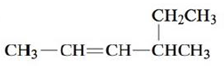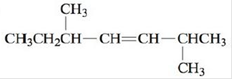Chapter 22, Problem 25E

Chapter
Section
Textbook Problem

# Name each of the following alkenes.a. CH2 = CH — CH2 — CH3b.c.(a)

Interpretation Introduction

Interpretation: The given alkenes are to be named.

Concept introduction: Rules given by IUPAC should be followed to name an organic compound. Any organic compound that has only one name denotes that compound. The root word determines the number of carbons while counting the longest carbon chain. Double of triple bond should be given lowest carbon number. If more than one substituent are present, prefixes like di, tri, tetra, etc. are used.

To determine: The name of the given alkene.

Explanation

Explanation

The given compound is,

Figure 1

Since, there are four carbons present...

(b)

Interpretation Introduction

Interpretation: The given alkenes are to be named.

Concept introduction: Rules given by IUPAC should be followed to name an organic compound. Any organic compound that has only one name denotes that compound. The root word determines the number of carbons while counting the longest carbon chain. Double of triple bond should be given lowest carbon number. If more than one substituent are present, prefixes like di, tri, tetra, etc. are used.

To determine: The name of the given alkene.

(c)

Interpretation Introduction

Interpretation: The given alkenes are to be named.

Concept introduction: Rules given by IUPAC should be followed to name an organic compound. Any organic compound that has only one name denotes that compound. The root word determines the number of carbons while counting the longest carbon chain. Double of triple bond should be given lowest carbon number. If more than one substituent are present, prefixes like di, tri, tetra, etc. are used.

To determine: The name of the given alkene.

### Still sussing out bartleby?

Check out a sample textbook solution.

See a sample solution

#### The Solution to Your Study Problems

Bartleby provides explanations to thousands of textbook problems written by our experts, many with advanced degrees!

Get Started